# Synchronous Motor (Part - 1) Electrical Engineering (EE) Notes | EduRev

## Electrical Engineering (EE) : Synchronous Motor (Part - 1) Electrical Engineering (EE) Notes | EduRev

The document Synchronous Motor (Part - 1) Electrical Engineering (EE) Notes | EduRev is a part of the Electrical Engineering (EE) Course Electrical Machines.
All you need of Electrical Engineering (EE) at this link: Electrical Engineering (EE)

Principle of operation

In order to understand the principle of operation of a synchronous motor, let us examine what happens if we connect the armature winding (laid out in the stator) of a 3-phase synchronous machine to a suitable balanced 3-phase source and the ﬁeld winding to a D.C source of appropriate voltage. The current ﬂowing through the ﬁeld coils will set up stationary magnetic poles of alternate North and South. ( for convenience let us assume a salient pole rotor, as shown in Fig. 50). On the other hand, the 3-phase currents ﬂowing in the armature winding produce a rotating magnetic ﬁeld rotating at synchronous speed. In other words there will be moving North and South poles established in the stator due to the 3-phase currents i.e at any location in the stator there will be a North pole at some instant of time and it will become a South pole after a time period corresponding to half a cycle. (after a time = 21f , where f = frequency of the supply). Let us assume that the stationary South pole in the rotor is aligned with the North pole in the stator moving in clockwise direction at a particular instant of time, as shown in Fig. 50. These two poles get attracted and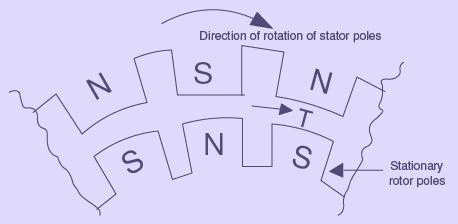Figure 50: Force of attraction between stator poles and rotor poles - resulting in production of torque in clockwise direction
try to maintain this alignment ( as per lenz’s law) and hence the rotor pole tries to follow the stator pole as the conditions are suitable for the production of torque in the clockwise direction. However the rotor cannot move instantaneously due to its mechanical inertia, and so it needs sometime to move. In the mean time, the stator pole would quickly (a time duration corresponding to half a cycle) change its polarity and becomes a South pole. So the force of attraction will no longer be present and instead the like poles experience a force of repulsion as shown in Fig. 51. In other words, the conditions are now suitable for theFigure 51: Force of repulsion between stator poles and rotor poles - resulting in production of torque in anticlockwise direction

production of torque in the anticlockwise direction. Even this condition will not last longer as the stator pole would again change to North pole after a time of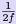. Thus the rotor will experience an alternating force which tries to move it clockwise and anticlockwise at twice the frequency of the supply, i.e. at intervals corresponding toseconds. As this duration is quite small compared to the mechanical time constant of the rotor, the rotor cannot respond and move in any direction. The rotor continues to be stationary only.

On the contrary if the rotor is brought to near synchronous speed by some external means say a small motor (known as pony motor-which could be a D.C or AC induction rotor) mounted on the same shaft as that of the rotor, the rotor poles get locked to the unlike poles in the stator and the rotor continues to run at the synchronous speed even if the supply to the pony motor is disconnected.

Thus the synchronous rotor cannot start rotating on its own or usually we say that the synchronous rotor has no starting torque. So, some special provision has to be made either inside the machine or outside of the machine so that the rotor is brought to near about its synchronous speed. At that time, if the armature is supplied with electrical power, the rotor can pull into step and continue to operate at its synchronous speed. Some of the commonly used methods for starting synchronous rotor are described in the following section.

Methods of starting synchronous motor

Basically there are three methods that are used to start a synchronous motor:

• To reduce the speed of the rotating magnetic ﬁeld of the stator to a low enough value that the rotor can easily accelerate and lock in with it during one half-cycle of the rotating magnetic ﬁeld’s rotation. This is done by reducing the frequency of the applied electric power. This method is usually followed in the case of inverter-fed synchronous motor operating under variable speed drive applications.
• To use an external prime mover to accelerate the rotor of synchronous motor near to its synchronous speed and then supply the rotor as well as stator. Ofcourse care should be taken to ensure that the direction of rotation of the rotor as well as that of the rotating magnetic ﬁeld of the stator are the same. This method is usually followed in the laboratory- the synchronous machine is started as a generator and is then connected to the supply mains by following the synchronization or paralleling procedure. Then the power supply to the prime mover is disconnected so that the synchronous machine will continue to operate as a motor.
• To use damper windings or amortisseur windings if these are provided in the machine. The damper windings or amortisseur windings are provided in most of the large synchronous motors in order to nullify the oscillations of the rotor whenever the synchronous machine is sub jected to a periodically varying load.

Each of these methods of starting a synchronous motor are described below in detail.

Motor Starting by Reducing the supply Frequency

If the rotating magnetic ﬁeld of the stator in a synchronous motor rotates at a low enough speed, there will be no problem for the rotor to accelerate and to lock in with the stator’s magnetic ﬁeld. The speed of the stator magnetic ﬁeld can then be increased to its rated operating speed by gradually increasing the supply frequency f up to its normal 50- or 60-Hz value.
This approach to starting of synchronous motors makes a lot of sense, but there is a big problem: Where from can we get the variable frequency supply? The usual power supply systems generally regulate the frequency to be 50 or 60 Hz as the case may be. However, variable-frequency voltage source can be obtained from a dedicated generator only in the olden days and such a situation was obviously impractical except for very unusual or special drive applications.
But the present day solid state power converters oﬀer an easy solution to this. We now have the rectiﬁer- inverter and cycloconverters, which can be used to convert a constant frequency AC supply to a variable frequency AC supply. With the development of such modern solid-state variable-frequency drive packages, it is thus possible to continuously control the frequency of the supply connected to the synchronous motor all the way from a fraction of a hertz up to and even above the normal rated frequency. If such a variable-frequency drive unit is included in a motor-control circuit to achieve speed control, then starting the synchronous motor is very easy-simply adjust the frequency to a very low value for starting, and then raise it up to the desired operating frequency for normal running.
When a synchronous motor is operated at a speed lower than the rated speed, its internal generated voltage (usually called the counter EMF) EA = K φω will be smaller than normal.
As such the terminal voltage applied to the motor must be reduced proportionally with the frequency in order to keep the stator current within the rated value. Generally, the voltage in any variable-frequency power supply varies roughly linearly with the output frequency.

Motor Starting with an External Motor

The second method of starting a synchronous motor is to attach an external starting motor (pony motor) to it and bring the synchronous machine to near about its rated speed (but not exactly equal to it, as the synchronization process may fail to indicate the point of closure of the main switch connecting the synchronous machine to the supply system) with the pony motor. Then the output of the synchronous machine can be synchronised or paralleled with its power supply system as a generator, and the pony motor can be detached from the shaft of the machine or the supply to the pony motor can be disconnected. Once the pony motor is turned OFF, the shaft of the machine slows down, the speed of the rotor magnetic ﬁeld BR falls behind Bnet, momentarily and the synchronous machine continues to operate as a motor. As soon as it begins to operates as a motor the synchronous motor can be loaded in the usual manner just like any motor.

This whole procedure is not as cumbersome as it sounds, since many synchronous motors are parts of motor-generator sets, and the synchronous machine in the motor-generator set may be started with the other machine serving as the starting motor. More over, the starting motor is required to overcome only the mechanical inertia of the synchronous machine without any mechanical load ( load is attached only after the synchronous machine is paralleled to the power supply system). Since only the motor’s inertia must be overcome, the starting motor can have a much smaller rating than the synchronous motor it is going to start. Generally most of the large synchronous motors have brushless excitation systems mounted on their shafts. It is then possible to use these exciters as the starting motors. For many medium-size to large synchronous motors, an external starting motor or starting by using the exciter may be the only possible solution, because the power systems they are tied to may not be able to handle the starting currents needed to use the damper (amortisseur) winding approach described next.

Motor Starting by Using damper (Amortisseur) Winding

As already mentioned earlier most of the large synchronous motors are provided with damper windings, in order to nullify the oscillations of the rotor whenever the synchronous machine is sub jected to a periodically varying load. Damper windings are special bars laid into slots cut in the pole face of a synchronous machine and then shorted out on each end by a large shorting ring, similar to the squirrel cage rotor bars. A pole face with a set of damper windings is shown in Figure..

When the stator of such a synchronous machine is connected to the 3-Phase AC supply, the machine starts as a 3-Phase induction machine due to the presence of the damper bars, just like a squirrel cage induction motor. Just as in the case of a 3-Phase squirrel cage induction motor, the applied voltage must be suitably reduced so as to limit the starting current to the safe rated value. Once the motor picks up to a speed near about its synchronous speed, the DC supply to its ﬁeld winding is connected and the synchronous motor pulls into step i.e. it continues to operate as a Synchronous motor running at its synchronous speed.

Behavior of a synchronous motor

The behavior of a synchronous motor can be predicted by considering its equivalent circuit on similar lines to that of a synchronous generator as described below.

Equivalent circuit model and phasor diagram of a synchronous motor

The equivalent-circuit model for one armature phase of a cylindrical rotor three phase synchronous motor is shown in Fig. 52 exactly similar to that of a synchronous generator except that the current ﬂows in to the armature from the supply. All values are given per phase.
Applying Kirchhoﬀ ’s voltage law to Fig. 52,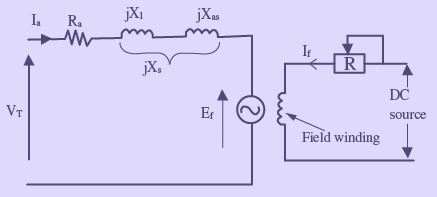Figure 52: Equivalent-circuit model for one phase of a synchronous motor armature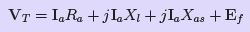(58)
Combining reactances, we have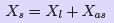(59)
Substituting Eqn. 59 in Eqn. 58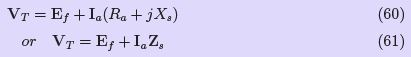where: Ra = armature resistance (Ω/phase)
X= armature leakage reactance (Ω/phase)
Xs = synchronous reactance (Ω/phase)
Zs = synchronous impedance (Ω/phase)
V= applied voltage/phase (V)
Ia = armature current/phase(A)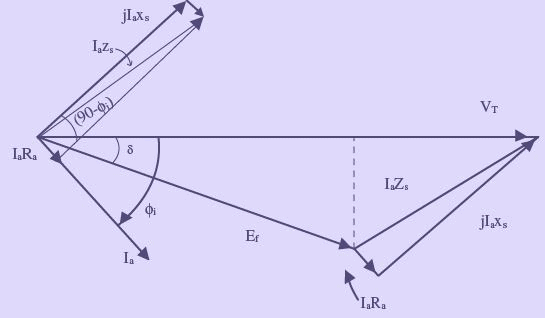Figure 53: Phasor diagram corresponding to the equivalent-circuit model

A phasor diagram shown in Fig. 53, illustrates the method of determining the counter EMF which is obtained from the phasor equation;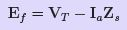The phase angle δ between the terminal voltage VT and the excitation voltage Ein Fig. 53 is usually termed the torque angle. The torque angle is also called the load angle or power angle.

Offer running on EduRev: Apply code STAYHOME200 to get INR 200 off on our premium plan EduRev Infinity!

66 docs|20 tests

,

,

,

,

,

,

,

,

,

,

,

,

,

,

,

,

,

,

,

,

,

;